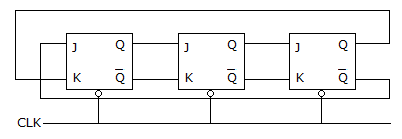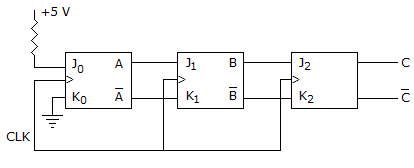# Test: Digital Electronics - 4

## 25 Questions MCQ Test Digital Electronics | Test: Digital Electronics - 4

Description
Attempt Test: Digital Electronics - 4 | 25 questions in 25 minutes | Mock test for Electrical Engineering (EE) preparation | Free important questions MCQ to study Digital Electronics for Electrical Engineering (EE) Exam | Download free PDF with solutions
QUESTION: 1

Solution:
QUESTION: 2

Solution:
QUESTION: 3

### Dynamic memory cells are constructed using

Solution:
QUESTION: 4

Which of the following is incorrect?

Solution:
QUESTION: 5

A 3 stage Johnson counter (ring) shown in figure is clocked at a constant frequency of fc from the starting state of Q0 Q1 Q2 = 101. The frequency of output Q0 Q1 Q2 will beSolution:
QUESTION: 6

A depletion type NMOS is operated in enhancement mode. Vp = - 4 volts. For VGS = + 3 volts as VDS is increased, ID becomes nearly constant when Vps equals

Solution:
QUESTION: 7

A number is expressed in binary 2's complement as 10011 decimal equivalent value is

Solution:
QUESTION: 8

Which one of the following is D/A conversion technique?

Solution:
QUESTION: 9

Assuming accumulator contain A 64 and the carry is set (1). What will accumulator (A) and carry (CY) contain after ANA A?

Solution:
QUESTION: 10

In INHIBIT operation

Solution:
QUESTION: 11

A JK flip flop can be converted to D flip flop by

Solution:
QUESTION: 12

The product of which of the following gives the figure of merit of a logic family?

Solution:
QUESTION: 13

In a 4 bit parallel in parallel out shift register A = 1, B = 1, C = 0, D = 1. The data output after 3 clock pulses is

Solution:
QUESTION: 14

Assertion (A): A 4 input variable logic circuit can be implemented using a 8 : 1 multiplexer.

Reason (R): When a multiplexer is used as a logic function generator, the logic design is simple.

Solution:
QUESTION: 15

A 6 MHz channel is used by a digital signalling system initializing four-level signals. The maximum possible transmission rate is

Solution:
QUESTION: 16

Precisely 1 K byte means

Solution:
QUESTION: 17

Data can be changed from spatial code to temporal code and vice-versa by using

Solution:
QUESTION: 18

In 8156, the lower times byte is addressed with 24 H, and the upper times byte with

Solution:
QUESTION: 19

In the CMOS inverter

Solution:
QUESTION: 20

Which of the following is incorrect?

Solution:
QUESTION: 21

Which one of the following is used to change data from spatial code to temporal code?

Solution:
QUESTION: 22

Which of the following needs DC forward voltage to emit light?

Solution:
QUESTION: 23

If a microcomputer has a 64 K memory; what is the hexadecimal notations for the last memory location?

Solution:
QUESTION: 24

In the given figure shows a 3 bit shift register using TTL flip flops. Initially all the flip flops are set to 0 state. After 8 clock pulsesSolution:
QUESTION: 25

Recommended fanout for ECL is

Solution:Use Code STAYHOME200 and get INR 200 additional OFF Use Coupon Code